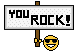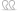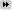1. ## Good question.

So there are a few smart people on here (more that a few smart arses).
I'm assuming they mean choose an answer from the 4 options.Reply With Quote

2. What question ?Reply With Quote

3. b.......Reply With Quote

4. If Psuedolous answered the question , the chances of him getting it right would be zero %Reply With Quote

5. There is no question.Reply With Quote

6. There is a question .Reply With Quote

7.Originally Posted by Fluke
There is a question .
What is it?Reply With Quote

8.Originally Posted by English NoodlesOriginally Posted by Fluke
There is a question .
What is it?
It is.

IF you choose an answer to this question at random (I'm assuming they mean from the 4 options given) WHAT is the chance you will be correct?
I'm thinking the correct answer is not among the options.Reply With Quote

9.Originally Posted by Necron99b.......
If B is correct there woukld only have been a 25% chance, not a 50% chance, so it can't be B.Reply With Quote

Assuming you may only choose one answer out of four, then your chances are 25%
But there are two answers of 25% then you would be randomly correct 50% of the time, making B the correct answer. But you would only choose B 25% of the time so it is incorrect...Reply With Quote

11. ^Yes, I just googled the maths forums too. There is no correct answer, in which case the possibility is 0%.

http://www.reddit.com/r/math/comment...nswer_to_this/Reply With Quote

12.Originally Posted by Fluke
If Psuedolous answered the question , the chances of him getting it right would be zero %
You really are fecking obsessed aren't you?

Oii Mid - where are you with your spam bot delete button?

Anyway, its a paradox, n'est pas?Originally Posted by English Noodles
There is no correct answer, in which case the possibility is 0%.
If one of the answers was 0% though, what would be the answer then?Reply With Quote

13.Originally Posted by pseudolus
If one of the answers was 0% though, what would be the answer then?
That question is actually answered in the thread I linked to. I can't be arsed to read through it again. It's in there though. Something to do with fixed point mathematics.Reply With Quote

14. If 0 was a choice and 0 was correct then there would be a 25% chance except that in that case 0 wouldn't be correct.

Take the blue pill. It's a Kobayashi Maru with a slight case of two weevils on the side.Reply With Quote

15. Ah no, Mao, If 0% was one of the answers, you'd then have a 37.5% chance of getting the right answer, but as 37.5% is not one of the answers, the answer is really 0%, but as we just found out it can not be 0% if 0% is one of the answers. .... ..its a paradox. If 37.5% was one of the answer, they that would be the answer.Reply With Quote

16.Originally Posted by pseudolus
If 37.5% was one of the answer, they that would be the answer.Reply With Quote

17. Here's one. Genuine question with a genuine answer...

Is this a question?Reply With Quote

18. Yes, if this is an answer.Reply With Quote

19.Originally Posted by English Noodles
Yes, if this is an answer.
Well done. You have passed the Oxford University entrance examination....Reply With Quote

20.Originally Posted by English Noodles
I just worked it out with a piece of paper though -so I have to read something? Can't you tell us ?Reply With Quote

21. What does "it" mean in the sentence "What time is it?"?Reply With Quote

22.Originally Posted by pseudolus
I just worked it out with a piece of paper though -so I have to read something? Can't you tell us ?
No, you can read for yourself.Reply With Quote

23.Originally Posted by pseudolusOriginally Posted by English Noodles
I just worked it out with a piece of paper though -so I have to read something? Can't you tell us ?
You needed a piece of paper?Reply With Quote

24. If you have 2 correct answers in a self-referencing 4-way mutually exclusive multiple choice question then the probability of choosing the correct answer is 0% so the correct answer does not appear in the option list in this case.Reply With Quote

25. Ignore the a, b, c, d and its quite obvious its an unanswerable question

Edit,, you thick kuntsReply With Quote